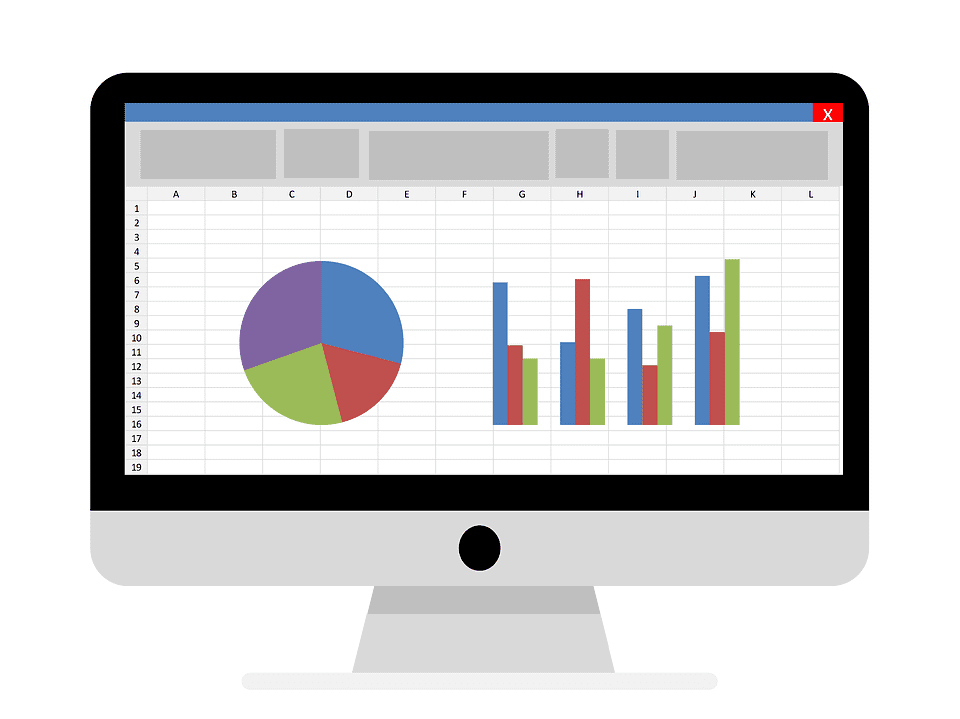# Statistics and Probability – A General Introduction

## Statistics and Probability – A General Introduction

Statistics and probability are branches of mathematics that focus on data collection and analysis. Probability is defined as the state or quality of being probable; or the extent to which an event is likely to occur.

For example, you can say that destruction and loss of life are high probabilities when a high-category hurricane hits the mainland.

## What Is Statistics All About?

Statistics is the process by which data is collected and analyzed in large quantities – and this is mainly done for purposes of inferring distinct proportions in a larger whole by using a sample as a representative. For example, you can say that statistics show an improvement in job prospects for new graduates.

A typical statistical representation involves the collection of data, thorough analysis, organization, and presentation. When using statistical data to present a mathematical problem, it is common to start with a statistical model or population for additional study. This “population” can be anything such as the total number of people living in a particular country, or the total number of government sedans in distribution in a particular state.

Census data is also collected for purposes of presenting statistical information; however when this isn’t possible, a statistician may use survey samples or experimental designs for the same purpose.

## What Is Probability All About?

Probability, on the other hand, is quantified as a distinct number between 0 (zero), and 1 (one). In this case, the zero represents total unlikeliness of an occurrence, and number one represents certainty that an event will occur. Generally speaking, the higher the probability of any event, the more likely that it will happen.

As an example:

Consider the case of a simple coin toss:
When the coin is in the air, the outcome has equal chances of being either heads or tails (because the coin isn’t biased); and in this case both outcomes are equally probable (i.e., 0.5, or 50% chance).

When using probability in general experiments like the coin toss, it is conventional to describe each probability numerically by incorporating the number of desired outcomes and dividing that number with the complete number of possible outcomes.

## Probability Today

The modern study of probability has evolved over many millennia. Gambling is an old practice that helped to raise curiosity and interest in quantifying factors that influence probability – however the actual mathematics came about in recent times.

It is not without reason that for a long time the development of probability was stalled. Even with the interest spurred by games of chance, there was still an overshadowing preoccupation with luck and superstition; and these played a role in mystifying the actual mathematics.

Modern day application of statistics and probability include physics, modeling and risk assessment. Insurance and trading markets also use statistics to form their own theories. Governments and financial institutions also rely on probability and statistics to form the basis for important calculations.

For students interested in pursuing probability and statistics, there are college-level courses that take a closer look at select topics like basic combinations, hypothesis testing, linear regression, Bayesian inference, and confidence intervals.

Statistics and probability cover too many topics to list
them all here. To give you a wider understanding of all these topics, you will be provided plenty of learning and assessment tools including online problem sets, tutorial videos, and reading questions in any one of our fundamental courses in finance.

If you are interested in working in the financial markets or you want to start running your own portfolio then this site is the best place to start.

Start Here:

1.

2.    Learning The Stock Market

3.